# Generalization in Neural Networks

When training a neural network in deep learning, its performance on processing new data is key. Improving the model's ability to generalize relies on preventing overfitting using these important methods.

By Harsha Bommana, Datakalp | Deep Learning Demystified.

Whenever we train our own neural networks, we need to take care of something called the generalization of the neural network. This essentially means how good our model is at learning from the given data and applying the learnt information elsewhere.

When training a neural network, there’s going to be some data that the neural network trains on, and there’s going to be some data reserved for checking the performance of the neural network. If the neural network performs well on the data which it has not trained on, we can say it has generalized well on the given data. Let’s understand this with an example.

Suppose we are training a neural network which should tell us if a given image has a dog or not. Let’s assume we have several pictures of dogs, each dog belonging to a certain breed, and there are 12 total breeds within those pictures. I’m going to keep all the images of 10 breeds of dogs for training, and the remaining images of the 2 breeds will be kept aside for now.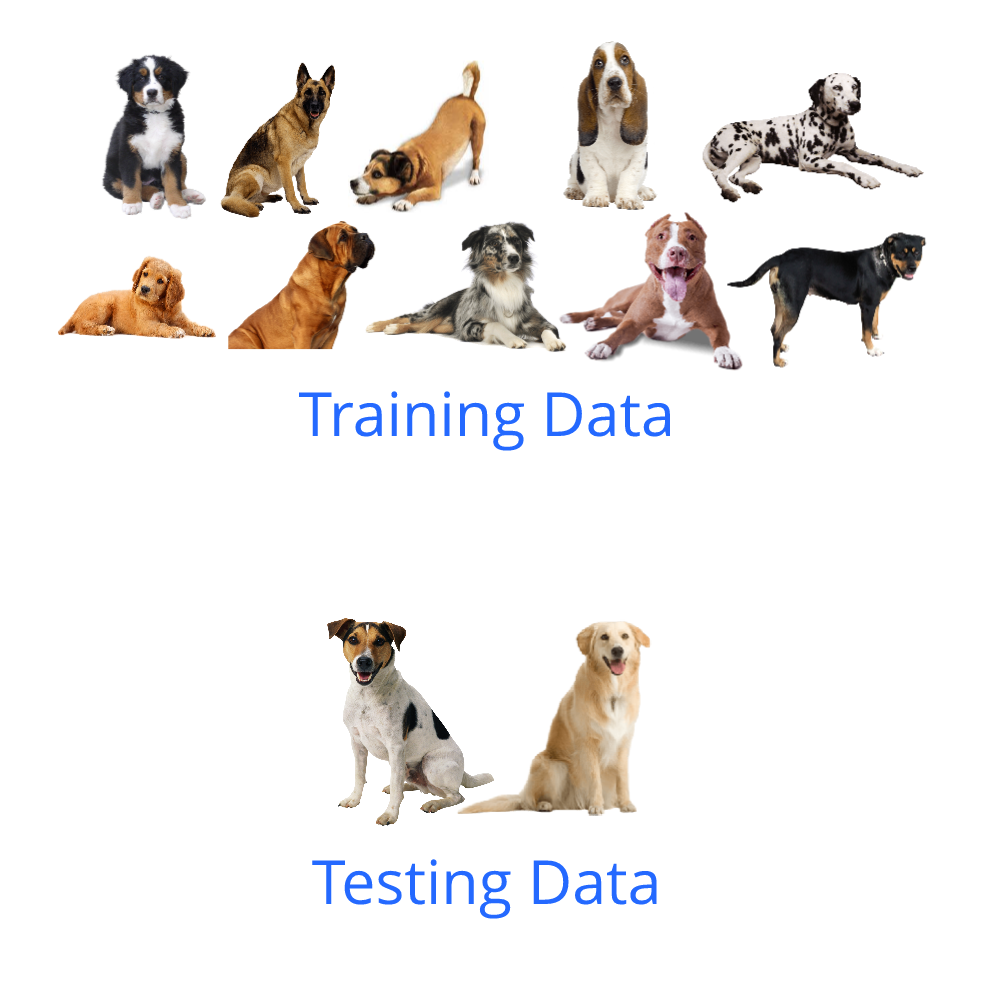Dogs training testing data split.

Now before going to the deep learning side of things, let’s look at this from a human perspective. Let’s consider a human being who has never seen a dog in their entire life (just for the sake of an example). Now we will show this human the 10 breeds of dogs and tell them that these are dogs. After this, if we show them the other 2 breeds, will they be able to tell that they are also dogs? Well hopefully they should, 10 breeds should be enough to understand and identify the unique features of a dog. This concept of learning from some data and correctly applying the gained knowledge on other data is called generalization.

Coming back to deep learning, our aim is to make the neural network learn as effectively from the given data as possible. If we successfully make the neural network understand that the other 2 breeds are also dogs, then we have trained a very general neural network, and it will perform really well in the real world.

This is actually easier said than done, and training a general neural network is one of the most frustrating tasks of a deep learning practitioner. This is because of a phenomenon in neural networks called overfitting. If the neural network trains on the 10 breeds of dogs and refuses to classify the other 2 breeds of dogs as dogs, then this neural network has overfitted on the training data. What this means is that the neural network has memorized those 10 breeds of dogs and considers only them to be dogs. Due to this, it fails to form a general understanding of what dogs look like. Combating this issue while training Neural Networks is what we are going to be looking at in this article.

Now we don’t actually have the liberty to divide all our data on a basis like breed. Instead, we will simply split all the data. One part of the data, usually the bigger part (around 80–90%), will be used for training the model, and the rest will be used to test it. Our objective is to make sure that the performance on the testing data is around the same as the performance on the training data. We use metrics like loss and accuracy to measure this performance.

There are certain aspects of neural networks that we can control in order to prevent overfitting. Let’s go through them one by one. The first thing is the number of parameters.

### Number of Parameters

In a neural network, the number of parameters essentially means the number of weights. This is going to be directly proportional to the number of layers and the number of neurons in each layer. The relationship between the number of parameters and overfitting is as follows: the more the parameters, the more the chance of overfitting. I’ll explain why.

We need to define our problem in terms of complexity. A very complex dataset would require a very complex function to successfully understand and represent it. Mathematically speaking, we can somewhat associate complexity with non-linearity. Let’s recall the neural network formula.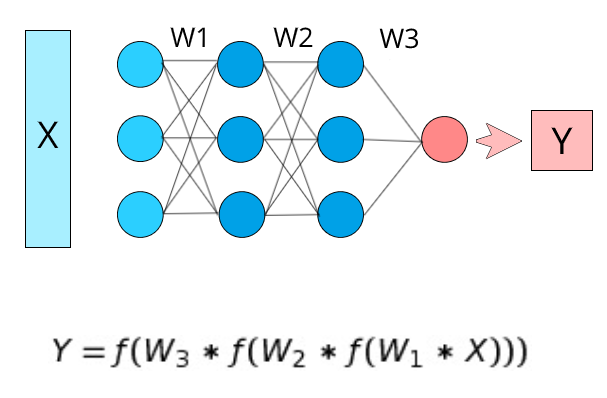Here, W1, W2, and W3 are the weight matrices of this neural network. Now what we need to pay attention to is the activation functions in the equation, which is applied to every layer. Because of these activation functions, each layer is nonlinearly connected with the next layer.

The output of the first layer is f(W_1*X) (Let it be L1), the output of the second layer is f(W_2*L1). As you can see here, because of the activation function (f), the output of the second layer has a nonlinear relationship with the first layer. So at the end of the neural network, the final value Y will have a certain degree of nonlinearity with respect to the input X depending on the number of layers in the neural network.

The more the number of layers, the more the number of activation functions disrupting the linearity between the layers, and hence the more the nonlinearity.

Because of this relationship, we can say that our neural network becomes more complex if it has more layers and more nodes in each layer. Hence we need to adjust our parameters based on the complexity of our data. There is no definite way of doing this except for repeated experimentation and comparing results.

In a given experiment, if the test score is much lower than the training score, then the model has overfit, and that means the neural network has too many parameters for the given data. This basically means that the neural network is too complex for the given data and needs to be simplified. If the test score is around the same as the training score, then the model has generalized, but this does not mean that we have reached the maximum potential of neural networks. If we increase the parameters the performance will increase, but it also might overfit. So we need to keep experimenting to optimize the number of parameters by balancing performance with generalization.

We need to match the neural network’s complexity with our data complexity. If the neural network is too complex, it will start memorizing the training data instead of having a general understanding of the data, hence causing overfitting.

Usually how deep learning practitioners go about this is to first train a neural network with a sufficiently high number of parameters such that the model will overfit. So initially, we try to get a model that fits extremely well on the training data. Next we try and reduce the number of parameters iteratively until the model stops overfitting, this can be considered as an optimal neural network. Another technique that we can use to prevent overfitting is using dropout neurons.

### Dropout Neurons

In neural networks, adding dropout neurons is one of the most popular and effective ways to reduce overfitting in neural networks. What happens in dropout is that essentially each neuron in the network has a certain probability of completely dropping out from the network. This means that at a particular instant, there will be certain neurons that will not be connected to any other neuron in the network. Here’s a visual example: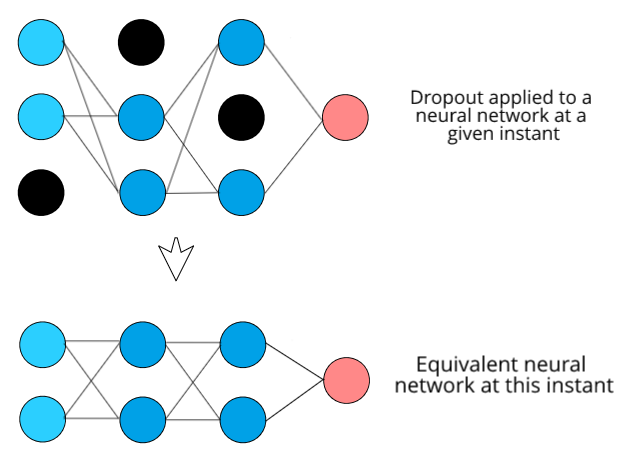At every instant during training, a different set of neurons will be dropped out in a random fashion. Hence we can say that at each instant we are effectively training a certain subset neural network that has fewer neurons than the original neural network. This subset neural network will change each and every time because of the random nature of the dropout neurons.

What essentially happens here is that while we train a neural network with dropout neurons, we are basically training many smaller subset neural networks and since the weights are a part of the original neural network, the final weights of the neural network can be considered as an average of all the corresponding subset neural network weights. Here’s a basic visualization of what’s going on: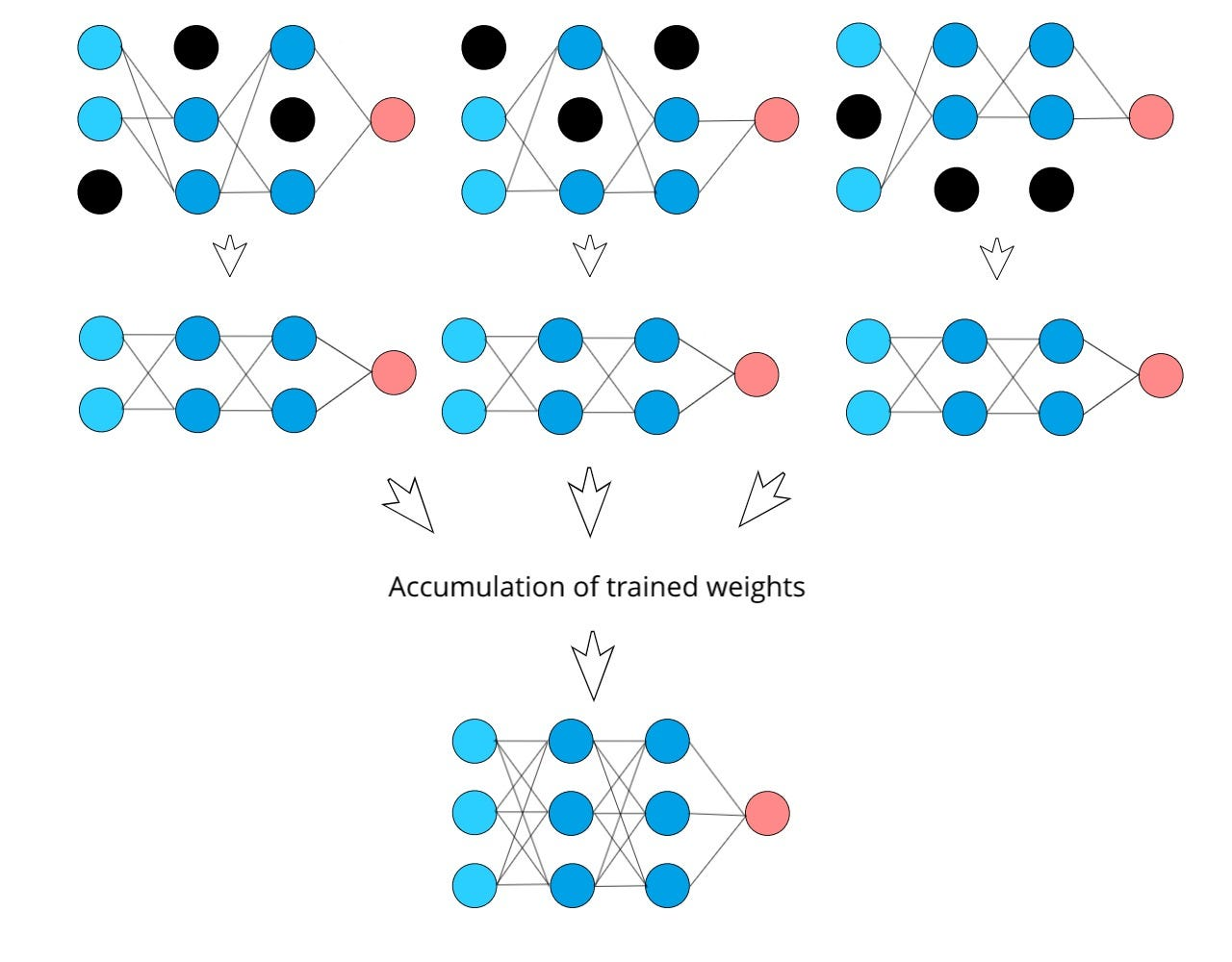This is how dropout neurons work in a neural network, but why does dropout prevent overfitting? There are two main reasons for this.

The first reason is that dropout neurons promote neuron independence. Because of the fact that the neurons surrounding a particular neuron may or may not exist during a certain instant, that neuron cannot rely on those neurons which surround it. Hence it will be forced to be more independent while training.

The second reason is that because of dropout, we are essentially training multiple smaller neural networks at once. In general, if we train multiple models and average their weights, the performance usually increases because of the accumulation of independent learnings of each neural network. However, this is an expensive process since we need to define multiple neural networks and train them individually. However, in the case of dropout, this does the same thing while we need only one neural network from which we are training multiple possible configurations of subset neural networks.

Training multiple neural networks and aggregating their learnings is called “ensembling” and usually boosts performance. Using dropout essentially does this with while having only 1 neural network.

The next technique for reducing overfitting is weight regularization.

### Weight Regularization

While training neural networks, there is a possibility that the value of certain weights can become very large. This happens because these weights are focusing on certain features in the training data, which is causing them to increase in value continuously throughout the training process. Because of this, the network overfits on the training data.

We don’t need the weight to continuously increase to capture a certain pattern. Instead, it’s fine if they have a value that is higher than the other weights on a relative basis. But during the training process while a neural network is trained on the data over multiple iterations, the weights have a possibility of constantly increasing in value till they become huge, which is unnecessary.

One of the other reasons why huge weights are bad for a neural network is because of the increased input-output variance. Basically when there is a huge weight in the network, it is very susceptible to small changes in the input, but the neural network should essentially output the same thing for similar inputs. When we have huge weights, even when we keep two separate data inputs, that are very similar, there is a chance that the outputs vastly differ. This causes many incorrect predictions to occur on the testing data, hence decreasing the generalization of the neural network.

The general rule of weights in neural networks is that the higher the weights in the neural network, the more complex the neural network. Because of this, neural networks having higher weights generally tend to overfit.

So basically, we need to limit the growth of the weights so that they don’t grow too much, but how exactly do we go about this? Neural networks try to minimize the loss while training, so we can try to include a part of the weights in that loss function so that weights are also minimized while training, but of course decreasing the loss in the first priority.

There are two methods of doing this called the L1 and L2 regularization. In L1 we take a small part of the sum of all the absolute values of the weights in the network. In L2, we take a small part of the sum of all the squared values of the weights in the network. We just add this expression to the overall loss function of the neural network. The equations are as follows: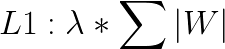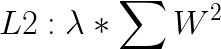Here, lambda is a value that allows us to alter the extent of weight change. We basically just add the L1 or L2 terms to the loss function of the neural net so that the network will also try to minimize these terms. By adding L1 or L2 regularization, the network will limit the growth of its weights since the magnitude of the weights are a part of the loss function, and the network always tries to minimize the loss function. Let’s highlight some of the differences between L1 and L2.

With L1 regularization, while a weight is decreasing due to regularization, L1 tries to push it down completely to zero. Hence unimportant weights that aren’t contributing much to the neural network will eventually become zero. However, in the case of L2, since the square function becomes inversely proportional for values below 1, the weights aren’t pushed to zero, but they are pushed to small values. Hence the unimportant weights have much lower values than the rest.

That covers the important methods of preventing overfitting. In deep learning, we usually use a mix of these methods to improve the performance of our neural networks and to improve the generalization of our models.

Original. Reposted with permission.

Related:

By subscribing you accept KDnuggets Privacy Policy

By subscribing you accept KDnuggets Privacy Policy

By subscribing you accept KDnuggets Privacy Policy